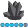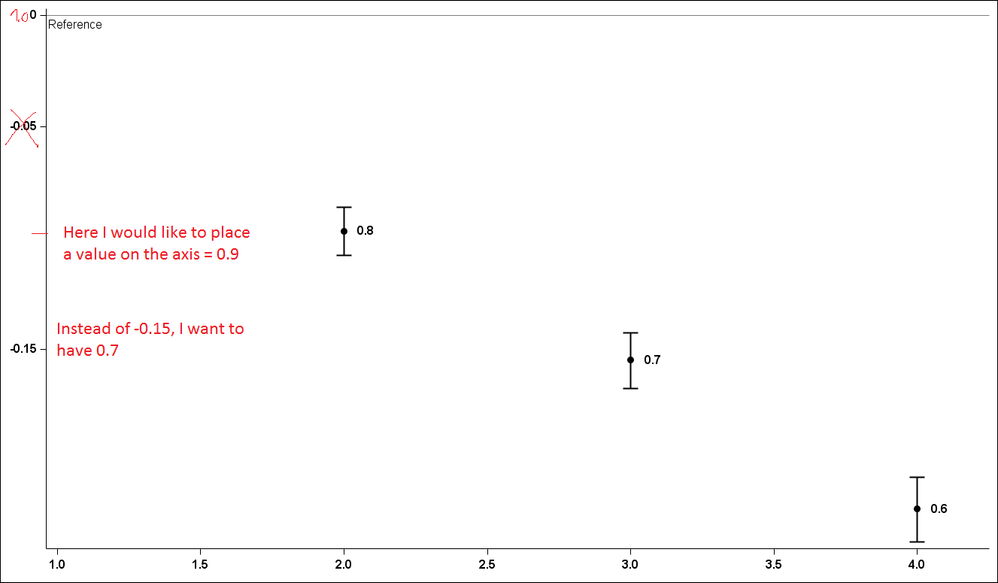AncaTilea
Pyrite | Level 9

## Linear values on a log-scale y-axis

Hi.

I have hazard ratios (HR) from a survival model. I want to plot the log of HR with the associated confidence interval, BUT display the LINEAR values.

I got the data labels to show the linear values, but I can't figure out how to modify the y-axis to plot the log values, but show the linear values.

Below is what I have, as an example,

data have;

input Group  HR Low High;

l_hr=log10(hr);

l_low=log10(low);

l_high=log10(high);

datalines;

1  .      .         .

2  0.80  0.78     0.82

3  0.70  0.68     0.72

4   0.60  0.58     0.62

;

run;

proc template;

define statgraph Graph;

dynamic _GROUP _L_HR _L_HIGH _L_LOW _HR;

begingraph / designwidth=1327 designheight=775;

layout lattice / rowdatarange=data columndatarange=data rowgutter=10 columngutter=10;

layout overlay / walldisplay=none xaxisopts=( display=(TICKS TICKVALUES  LINE )

tickvalueattrs=(size=12 style=NORMAL weight=BOLD ))

yaxisopts=( display=(TICKS TICKVALUES LINE )

tickvalueattrs=(size=12 style=NORMAL weight=BOLD)

linearopts=( /*tickvaluesequence=( start=-0.25 end=0.0 INCREMENT = 0.05)*/

tickvaluelist=(-0.25 -0.15 -0.05 0.00 )) logopts=( base=10));

scatterplot x=_GROUP y=_L_HR / datalabel=_HR yerrorupper=_L_HIGH yerrorlower=_L_LOW name='scatter'

datalabelposition=RIGHT datalabelattrs = (size = 12 WEIGHT = BOLD) markerattrs=(symbol=CIRCLEFILLED size=9 ) errorbarattrs=(thickness=2 );

referenceline y=0.0 / name='href' curvelabel = "Reference"

curvelabelposition = min curvelabelattrs = (size = 12) yaxis=Y;

endlayout;

endlayout;

endgraph;

end;

run;

proc sgrender data=WORK.have template=Graph;

dynamic _GROUP="GROUP" _L_HR="'L_HR'n" _L_HIGH="'L_HIGH'n" _L_LOW="'L_LOW'n" _HR="HR";

run;

Any suggestions are appreciated!

Thank you!

Anca.

PS: how can I paste SAS code in this compose box and make it look like SAS code (color coded)?1 ACCEPTED SOLUTION

Accepted SolutionsJay54
Meteorite | Level 14

## Re: Linear values on a log-scale y-axis

You are transforming the data itself into the log scale.  What if you keep the data as is, and set the Y axis to log?

2 REPLIES 2Jay54
Meteorite | Level 14

## Re: Linear values on a log-scale y-axis

You are transforming the data itself into the log scale.  What if you keep the data as is, and set the Y axis to log?AncaTilea
Pyrite | Level 9

## Re: Linear values on a log-scale y-axis

Thank you Sanjay,

I was transferring from old way of GPLOT + ANNO where I needed to use the logs...

Thanks.

Got it!

Anca.

Discussion stats
• 2 replies
• 1935 views
• 0 likes
• 2 in conversation